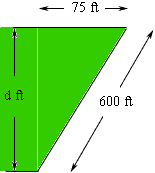Quandaries and Queries HELLO MY NAME IS DANA ,I AM AVERAGE JOE WITH A QUESTION THAT I CANNOT ANSWER MYSELF SO I CAME TO YOU, I HAVE A PIECE OF PROPERTY IN THE SHAPE OF AN ISOSCELES TRAPEZOID THAT IS 250 FEET ACROSS THE BACK, 100 FEET ACROSS THE FRONT AND BOTH SIDES ARE 600 FEET LONG.  HOW MANY SQUARE FEET ARE IN THIS PROPERTY.?I APPRECIATE YOUR HELP. THANK YOU DANA Hi Dana, In the answer to a previous question I showed that the area of a trapezoid is average of the lengths of the parallel sides times the distance between the parallel sides. In your lot the average of the lengths of the parallel sides is (100 + 250)/2 = 175 feet. You can use the symmetry of your lot and Pythagoras' theorem to find the distance between the parallel sides. Below is a diagram of one side of your lot.The 75 ft measurement I know by the symmetry and I have used d ft as the distance between the parallel sides. Pythagoras' Theorem tells me that d2 + 752 = 6002  Thus d2 = 6002 - 752 = 354375 so d = 595.29 feet. Thus the area of the lot is 175595.29 = 104,176 square feet. Penny Go to Math Central